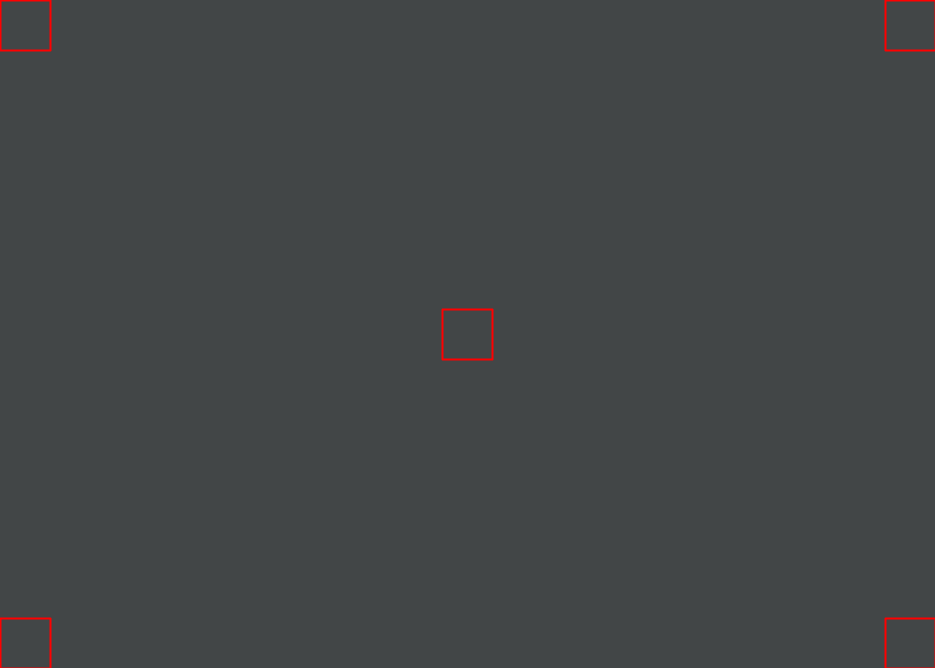## Canvas Bounds

This activity has the following desired goals:

• Learning about canvas bounds (A).
• Reviewing the x-y coordinate system (A).
• Using the above ideas to animate multiple objects and keeping them within the stage area (M, T).

### Step 1

Type in the following code and run it:

``````cleari()
// showAxes()
val cb = canvasBounds

val pic1 = Picture.rectangle(50, 100)
pic1.setPosition(cb.x + cb.width / 2, cb.y)

val pic2 = Picture.rectangle(100, 50)
pic2.setPosition(cb.x, cb.y + cb.height / 2)

draw(pic1, pic2)
``````

Q1a. What does the above code do? How does it do it?

Q1b. Is `pic1` perfectly centered in the horizontal directon at the bottom of the canvas?

Q1c. Is `pic2` perfectly centered in the vertical directon at the left edge of the canvas?

Q1d. Modify the code above so that `pic1` is perfectly centered horizontally at the bottom of the canvas, and `pic2` is perfectly centered veritcally at the left edge of the canvas.

### Self exploration

Play with the above code and try out different ideas as you see fit.

### Explanation

Query description:

• canvasBounds - returns the current bounds of the canvas. The returned bounds contain 4 pieces of information:
• `x` and `y`, which are the coordinates of the bottom-left corner of the canvas.
• `width` - the width of the canvas.
• `height` - the height of the canvas.

### Exercise

Write a program to make the following figure: